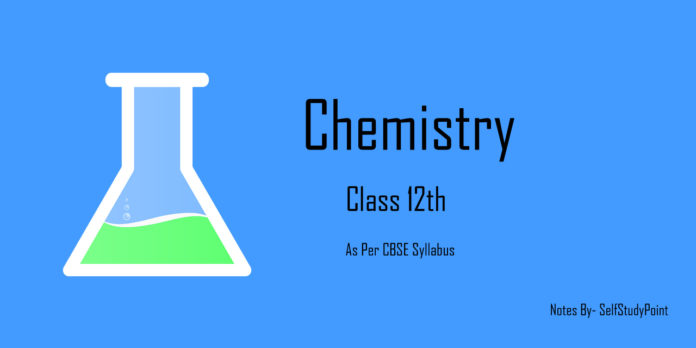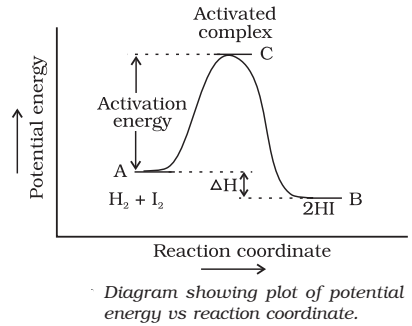# Temperature Dependency of a Rate of Reaction

## Activation Energy

According to collision theory, a reaction takes place because the reactant molecules collide with each other.

The minimum energy which the colliding molecules must have in order that the collision between them may be effective is called threshold energy.

The minimum extra amount of energy absorbed by the reactant molecules so that their energy becomes equal to the threshold value is called activation energy

Threshold energy = Activation energy + Energy possessed by the reactants

Less is the activation energy, faster is the reaction.

In order that the reactants may change into products, they have to cross an energy barrier (corresponding to threshold energy). Reactant molecules absorb energy and form an intermediate called activated complex which immediately dissociates to form the products.

Example:## Temperature Dependence of the Rate of a Reaction

For a chemical reaction with rise in temperature by 10°, the rate constant is nearly doubled.
Temperature coefficient = (Rate constant at T + 100) / (Rate constant at T0)

### Explanation:

At a particular temperature, if fractions of molecules are plotted versus corresponding kinetic energies, a graph of the type shown is obtained. The peak of the curve represents the kinetic energy possessed by the maximum fraction of molecules and is called most probable kinetic energy.With the increase in temperature:

• Maximum of the curve moves to the higher energy value i.e., most probable kinetic energy increases.
• The curve spreads to the right i.e., there is a greater proportion of molecules with much higher energies.